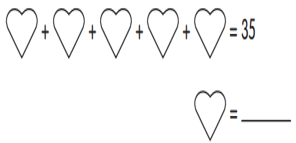RB 3rd Meaning of Multiplication
starstarstarstarstarstarstarstarstarstar
by SAMANTHA AYODELE
| 8 Questions
1
1
8 X 2= ____ + 10
A. 6
B. 8
C. 16
D. 18
2
1
We can put 20 peaches into ________.
A. 2 groups of 5
B. 2 groups of 10
C. 5 groups of 5
D. 5 groups of 103
1
How much does a heart equal?
A. 5
B. 6
C. 7
D. 8
4
1
Jonas saves \$2 every week.
He saves _____ in 9 weeks.5
1
Which number fits inside the box?6
1
Find the rule for this pattern. What is the missing number?7
1
Which picture shows 6x5?
A
B
C
D8
1
Zain likes to ride his bike. The table shows the number of days and the number of miles he rides in a week. How many miles does Zain ride his bike in a week? Write the multiplication equation that you can use to answer the question. _____ x ______= ______